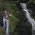## Tuesday, July 26, 2011Adding hyperlinks to a WPF RichTextBox can be achieved at design time in XAML or programmatically at run time.
Hyperlinks are created programmatically via the hyperlink constructor by parsing either the start and end TextPointers or InLine objects. These constructors are not particularly developer friendly unless the developer is programmatically populating a RichTextBox’s FlowDocument from Runs (see here).
In a recent project I need to add a hyperlink for text between predefined character index. For example, I needed a hyperlink for the text located between character positions 100 and 110.  The following extension method enables this.
RichTextBox extension:
```namespace ESRI.PrototypeLab.Sample {
public static class Extensions {
TextPointer p1 = rtb.ToTextPointer(start);
TextPointer p2 = rtb.ToTextPointer(end);
if (p1 == null || p2 == null) { return null; }
}
public static TextPointer ToTextPointer(this RichTextBox rtb, int index) {
int count = 0;
TextPointer position = rtb.Document.ContentStart;
while (position != null) {
if (position.GetPointerContext(LogicalDirection.Forward) ==
TextPointerContext.Text) {
string textRun = position.GetTextInRun(LogicalDirection.Forward);
int length = textRun.Length;
if (count + length > index) {
return position.GetPositionAtOffset(index - count);
}
count += length;
}
position = position.GetNextContextPosition(LogicalDirection.Forward);
}
return null;
}
}
}```
How to use:
```Hyperlink hyperlink = this.RichTextBox.ToHyperlink(100, 110);
hyperlink.Click += (s, e) => {
1.